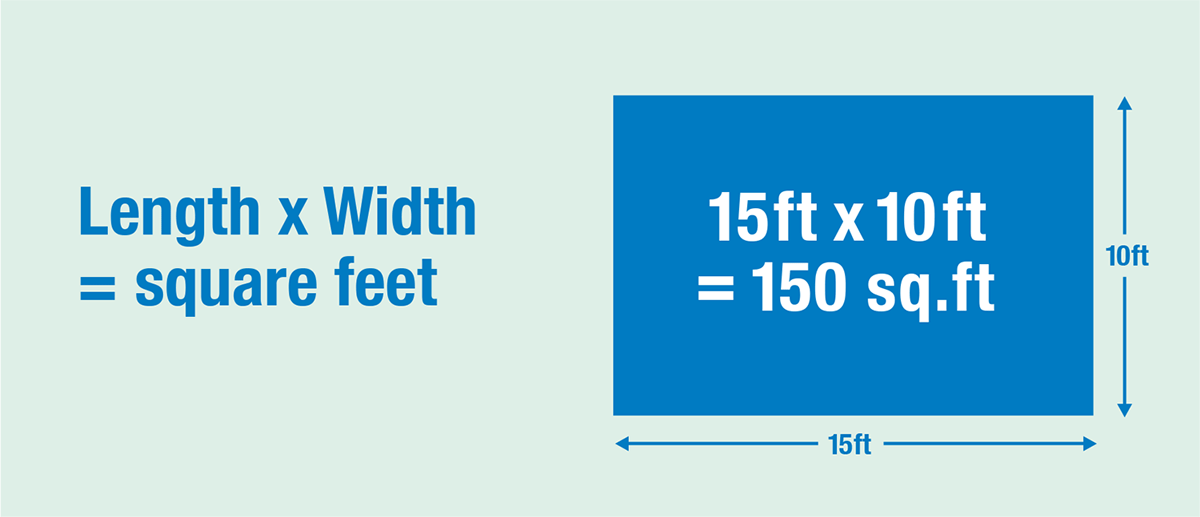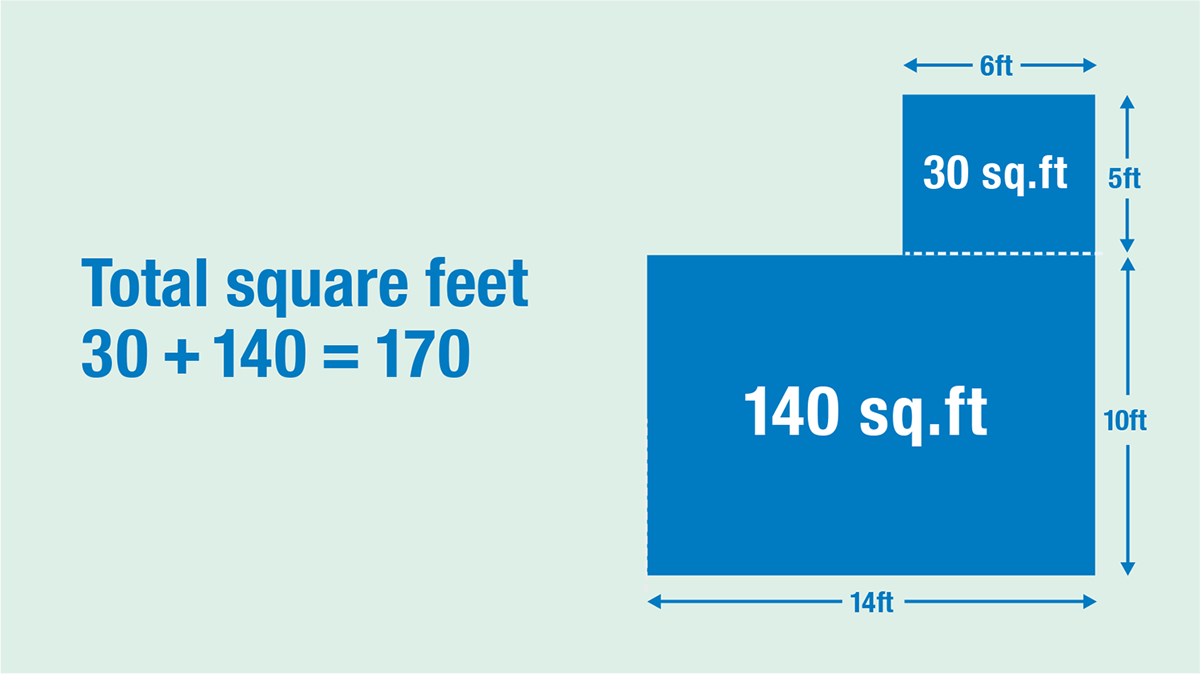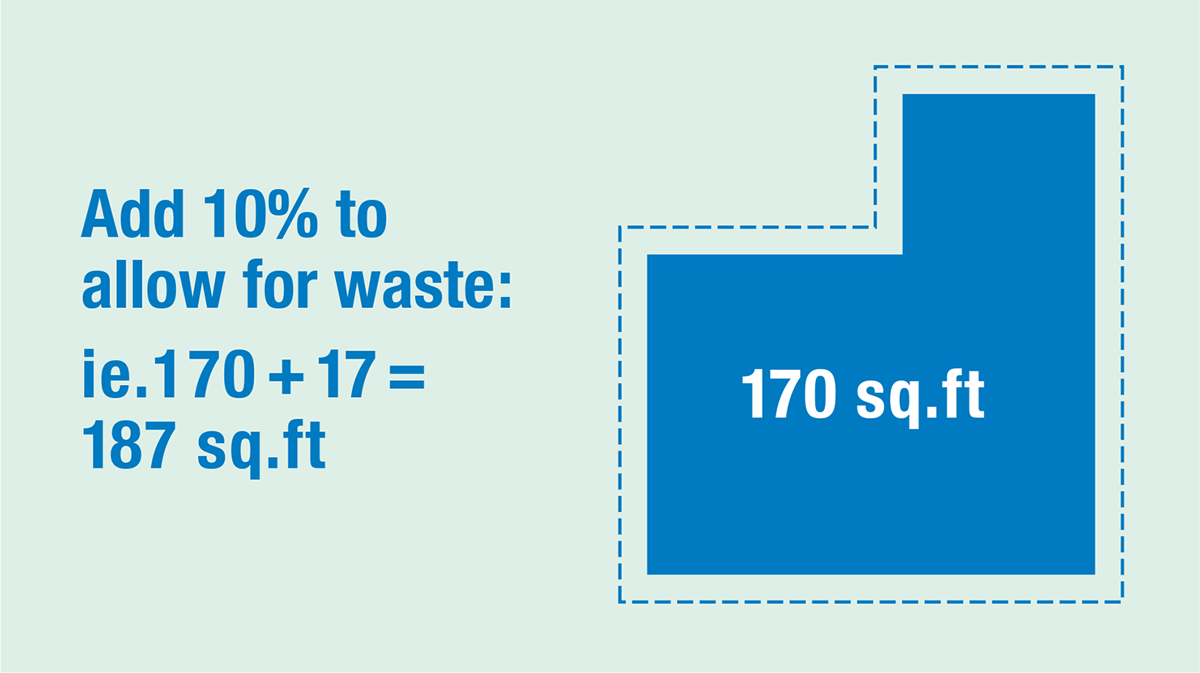# How to Calculate Square Footage - Step by Step

| Last update: 28 July 2023

Whether you're shopping for carpet, or planning a landscaping or home improvement project, one essential concept is calculating square footage. Join us as we discuss the steps involved in the process.

How to calculate the square footage of a room

Measure the length and width of the room in feet. Next, multiply the length and width together to get the square footage. Example: For a room measuring 10 feet wide by 14 feet long, your calculation is 10 × 14 = 140 square feet.

Having to work out a square footage calculation might give you panicky flashbacks of being late for school! But don't worry, our guide will show you to calculate square footage accurately. We also explain the formula for square footage, so you'll be able to ace any calculations that come up in your future projects, and we look at square feet calculations for irregular-shaped areas. And, as an extra-helpful bonus, we include a square footage calculator to help you check your calculations. Let's begin with step 1...

## 1 How to measure your room/spaceTo calculate the square feet of a rectangular area, you first need to measure two dimensions: the length and the width (note that we discuss irregular shaped areas further down).

• First, measure the longest side to find the length. Take a tape measure and fix one end of it to one end of the longest side. Note the measurement.
• Next, measure the width - the shortest side of the area to be calculated. Repeat the process above and note down that measurement.

## 2 Square footage formulaFor step 2, you're going to use the square footage formula:

Length × Width = Area (in square feet)

Note that the same formula applies if you measure in metric. In this case, the area will be expressed in square metres.

Insert your measurements into the formula. You're going to multiply the length measurement (in feet) by the width measurement. This gives you the area of the space in square feet.

You can also use this formula to calculate smaller areas when the area will be expressed in square inches and larger areas in square yards.

As an example, suppose you want to know how much flooring you might need to renovate a room that's 15 feet long × 10 feet wide. Multiply these dimensions:

15 ft × 10 ft = 150 sq. ft

## 3 Calculate irregular shapes

Sometimes, you might need to calculate a space that's an addition to the main area or a room that isn't evenly shaped. In these situations, think of the space as separate areas. You may find it helpful to sketch out a quick floor-plan to divide the space into more regular shapes.

• Measure the length and width of each separate area and calculate the area of each in square feet.
• Add your calculations to give you the total area in square feet.

### Let's look at an exampleSuppose you have a rectangular living room with a narrower dining area attached. Think of the space as being made up of a larger rectangle and a smaller rectangle.

First measure the length and width of the larger section labelled A, then do the same for the smaller section labelled B.

A: 14ft × 10 ft = 140 sq. ft
B: 6 ft × 5 ft = 30 sq. ft.

Now, add both values to calculate the square footage of the room:

140 sq.ft. + 30 sq.ft = 170 sq. ft

So you'll need 170 sq. ft. of flooring materials for this room.

### Triangular and circular shapes

It may be that the area you're measuring isn't square or rectangular, but triangular or circular. If this is the case we have a calculator for square footage that includes multiple room and area shapes. We also have instructions for calculating the square footage of a triangle and a circle. These include details of the measurements you will need to take.

### Measuring the area of a property

If you're selling a property yourself, you can measure the floor space of an entire home in the same way. Take the measurements of each room in turn, calculate the square footage of each, and then add all the values together to give the total square footage.

## 4 Use the square ft calculator

Check your calculations using our square footage calculator below.

ft in
ft in
ft in
ft in
ft in
Calculation results will appear here.Once you've calculated the square footage of the area, and know how much material you need to order for your project, adjust it to allow for waste. It's best to order 10% more than you think you'll need rather than the exact square footage.

Mistakes can happen, and if you only have the exact amount you need, you might not be able to source matching materials. If you find that you don't need the extra materials, suppliers are usually happy to refund unused tins of paint or unopened packs of tiles. And if you have space, it can be handy to store the surplus materials for any touch-up repairs that are needed in the future.

If you need to calculate the cost of materials for your project, simply multiply your total square feet figure by the price per square foot of the materials you're going to be using.

Once you've learned how to calculate the square footage of an area, you have a skill that will assist you in all your future renovation, landscaping and construction projects. And if time's short, you can use our handy online square footage calculator.